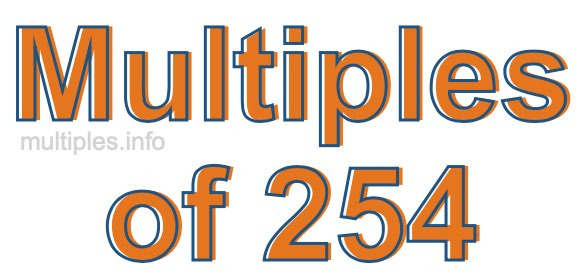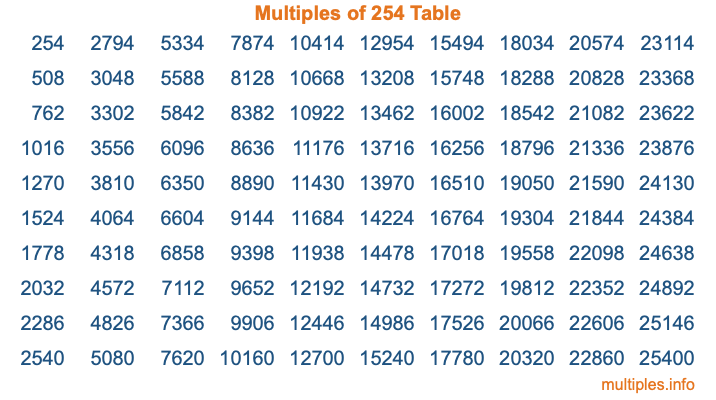Multiples of 254Welcome to the Multiples of 254 page. Here we will first teach you everything you will ever need to know about the multiples of 254, and then give you a study guide summary of everything we taught you to make sure you remember it all. Use this page to look up facts and learn information about the multiples of 254. This page will make you a multiples of two hundred fifty-four expert!

Definition of Multiples of 254
Multiples of 254 are all the numbers that when divided by 254 equal an integer. Each of the multiples of 254 are called a multiple. A multiple of 254 is created by multiplying 254 by an integer.

Therefore, to create a list of multiples of 254, you start with 1 multiplied by 254, then 2 multiplied by 254, then 3 multiplied by 254, and so on for as long as you want. Thus, the list of the first five multiples of 254 is 254, 508, 762, 1016, and 1270. To see a larger list of multiples of 254, see the printable image of Multiples of 254 further down on this page. We also have a category where you can choose any nth multiple of 254.

Multiples of 254 Checker
The Multiples of 254 Checker below checks to see if any number of your choice is a multiple of 254. In other words, it checks to see if there is any number (integer) that when multiplied by 254 will equal your number. To do that, we divide your number by 254. If the the quotient is an integer, then your number is a multiple of 254.

Is  a multiple of 254?

Least Common Multiple of 254 and ...
A Least Common Multiple (LCM) is the lowest multiple that two or more numbers have in common. This is also called the smallest common multiple or lowest common multiple and is useful to know when you are adding our subtracting fractions. Enter one or more numbers below (254 is already entered) to find the LCM.

Check out our LCM Calculator if you need more details about the Least Common Multiple or if you need the LCM for different numbers for adding and subtraction fractions.

nth Multiple of 254
As we stated above, 254 is the first multiple of 254, 508 is the second multiple of 254, 762 is the third multiple of 254, and so on. Enter a number below to find the nth multiple of 254.

th multiple of 254

Multiples of 254 vs Factors of 254
254 is a multiple of 254 and a factor of 254, but that is where the similarities end. All postive multiples of 254 are 254 or greater than 254. All positive factors of 254 are 254 or less than 254.

Below is the beginning list of multiples of 254 and the factors of 254 so you can compare:

Multiples of 254: 254, 508, 762, 1016, 1270, etc.

Factors of 254: 1, 2, 127, 254

As you can see, the multiples of 254 are all the numbers that you can divide by 254 to get a whole number. The factors of 254, on the other hand, are all the whole numbers that you can multiply by another whole number to get 254.

It's also interesting to note that if a number (x) is a factor of 254, then 254 will also be a multiple of that number (x).

Multiples of 254 vs Divisors of 254
The divisors of 254 are all the integers that 254 can be divided by evenly. Below is a list of the divisors of 254.

Divisors of 254: 1, 2, 127, 254

The interesting thing to note here is that if you take any multiple of 254 and divide it by a divisor of 254, you will see that the quotient is an integer.

Multiples of 254 Table
Below is an image of the first 100 multiples of 254 in a table. The table is in chronological order, column by column. The first column has the first ten multiples of 254, the second column has the next ten multiples of 254, and so on.The Multiples of 254 Table is also referred to as the 254 Times Table or Times Table of 254. You are welcome to print out our table for your studies.

Negative Multiples of 254
Although not often discussed or needed in math, it is worth mentioning that you can make a list of negative multiples of 254 by multiplying 254 by -1, then by -2, then by -3, and so on, to get the following list of negative multiples of 254:

-254, -508, -762, -1016, -1270, etc.

Multiples of 254 Summary
Below is a summary of important Multiples of 254 facts that we have discussed on this page. To retain the knowledge on this page, we recommend that you read through the summary and explain to yourself or a study partner why they hold true.

There are an infinite number of multiples of 254.

A multiple of 254 divided by 254 will equal a whole number.

254 divided by a factor of 254 equals a divisor of 254.

The nth multiple of 254 is n times 254.

The largest factor of 254 is equal to the first positive multiple of 254.

254 is a multiple of every factor of 254.

254 is a multiple of 254.

A multiple of 254 divided by a divisor of 254 equals an integer.

254 divided by a divisor of 254 equals a factor of 254.

Any integer times 254 will equal a multiple of 254.

Multiples of a Number
Here you can get the multiples of another number, all with the same attention to detail as we did for multiples of 254 on this page.

Multiples of
Multiples of 255
Did you find our page about multiples of two hundred fifty-four educational? Do you want more knowledge? Check out the multiples of the next number on our list!